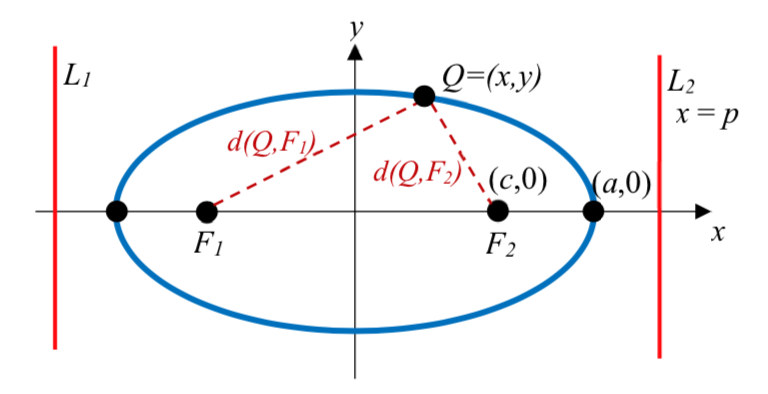$$\newcommand{\id}{\mathrm{id}}$$ $$\newcommand{\Span}{\mathrm{span}}$$ $$\newcommand{\kernel}{\mathrm{null}\,}$$ $$\newcommand{\range}{\mathrm{range}\,}$$ $$\newcommand{\RealPart}{\mathrm{Re}}$$ $$\newcommand{\ImaginaryPart}{\mathrm{Im}}$$ $$\newcommand{\Argument}{\mathrm{Arg}}$$ $$\newcommand{\norm}{\| #1 \|}$$ $$\newcommand{\inner}{\langle #1, #2 \rangle}$$ $$\newcommand{\Span}{\mathrm{span}}$$

# 9.4.4E: Conics in Polar Coordinates (Exercises)

$$\newcommand{\vecs}{\overset { \rightharpoonup} {\mathbf{#1}} }$$ $$\newcommand{\vecd}{\overset{-\!-\!\rightharpoonup}{\vphantom{a}\smash {#1}}}$$$$\newcommand{\id}{\mathrm{id}}$$ $$\newcommand{\Span}{\mathrm{span}}$$ $$\newcommand{\kernel}{\mathrm{null}\,}$$ $$\newcommand{\range}{\mathrm{range}\,}$$ $$\newcommand{\RealPart}{\mathrm{Re}}$$ $$\newcommand{\ImaginaryPart}{\mathrm{Im}}$$ $$\newcommand{\Argument}{\mathrm{Arg}}$$ $$\newcommand{\norm}{\| #1 \|}$$ $$\newcommand{\inner}{\langle #1, #2 \rangle}$$ $$\newcommand{\Span}{\mathrm{span}}$$ $$\newcommand{\id}{\mathrm{id}}$$ $$\newcommand{\Span}{\mathrm{span}}$$ $$\newcommand{\kernel}{\mathrm{null}\,}$$ $$\newcommand{\range}{\mathrm{range}\,}$$ $$\newcommand{\RealPart}{\mathrm{Re}}$$ $$\newcommand{\ImaginaryPart}{\mathrm{Im}}$$ $$\newcommand{\Argument}{\mathrm{Arg}}$$ $$\newcommand{\norm}{\| #1 \|}$$ $$\newcommand{\inner}{\langle #1, #2 \rangle}$$ $$\newcommand{\Span}{\mathrm{span}}$$

Section 9.4 Exercises

In problems 1–8, find the eccentricity and directrix, then identify the shape of the conic.

1. $$r = \dfrac{12}{1 + 3\cos (\theta )}$$

2. $$r = \dfrac{4}{1 - \sin (\theta )}$$

3. $$r = \dfrac{2}{4 - 3\sin (\theta )}$$

4. $$r = \dfrac{7}{2 - \cos (\theta )}$$

5. $$r = \dfrac{1}{5 - 5\cos (\theta )}$$

6. $$r = \dfrac{6}{3 + 8\cos (\theta )}$$

7. $$r = \dfrac{4}{7 + 2\cos (\theta )}$$

8. $$r = \dfrac{16}{4 + 3\sin (\theta )}$$

In problems 9–14, find a polar equation for a conic having a focus at the origin with the given characteristics.

9. Directrix $$x = -4$$, eccentricity $$e = 5$$.

10. Directrix $$y = -2$$, eccentricity $$e = 3$$.

11. Directrix $$y = 3$$, eccentricity $$e = \dfrac{1}{3}$$.

12. Directrix $$x = 5$$, eccentricity $$e = \dfrac{3}{4}$$.

13. Directrix $$y = -2$$, eccentricity $$e = 1$$.

14. Directrix $$x = -3$$, eccentricity $$e = 1$$.

In problems 15–20, sketch a graph of the conic. Use the graph to help you find important features and write a Cartesian equation for the conic.

15. $$r = \dfrac{9}{1 - 2\cos (\theta )}$$

16. $$r = \dfrac{4}{1 + 3\sin (\theta )}$$

17. $$r = \dfrac{12}{3 + \sin (\theta )}$$

18. $$r = \dfrac{15}{3 - 2\cos (\theta )}$$

19. $$r = \dfrac{6}{1 + \cos (\theta )}$$

20. $$r = \dfrac{4}{1 - \sin (\theta )}$$

21. At the beginning of the chapter, we defined an ellipse as the set of all points $$Q$$ for which the sum of the distance from each focus to $$Q$$ is constant. Mathematically, $$d\left( Q, F_1 \right) + d\left( Q, F_2 \right) = k$$. It is not obvious that this definition and the one provided in this section involving the directrix are related. In this exercise, we will start with the definition from this section and attempt to derive the earlier formula from it.

a. Draw an ellipse with foci at $$\left( c,0 \right)$$ and $$\left( - c,0 \right)$$, vertices at $$\left( a,0 \right)$$ and $$\left( - a,0 \right)$$, and directrixes at $$x = p$$ and $$x = - p$$. Label the foci $$F_1$$ and $$F_2$$. Label the directrixes $$L_1$$ and $$L_2$$. Label some point $$\left( x,y \right)$$ on the ellipse $$Q$$.
b. Find formulas for $$d\left( Q,L_1 \right)$$ and $$D\left( Q, L_2 \right)$$ in terms of $$x$$ and $$p$$.
c. From the definition of a conic in this section, $$\dfrac{d\left( Q,F_1 \right)}{d\left( Q,L_1 \right)} = e$$. Likewise, $$\dfrac{d\left( Q,F_2 \right)}{d\left( Q,L_2 \right)} = e$$ as well. Use these ratios, with your answers from part (b) above, to find formulas for $$d\left( Q, F_1 \right)$$ and $$D\left( Q, F_2 \right)$$ in terms of $$e$$, $$x$$, and $$p$$.
d. Show that the sum, $$d\left( Q, F_1 \right) + d\left( Q, F_2 \right)$$, is constant. This establishes that the definitions are connected.
e. Let $$Q$$ be a vertex. Find the distances $$d\left( Q, F_1 \right)$$ and $$D\left( Q, F_2 \right)$$ in terms of $$a$$ and $$c$$. Then combine this with your result from part (d) to find a formula for $$p$$ in terms of $$a$$ and $$e$$.
f. Let $$Q$$ be a vertex. Find the distances $$D\left( Q, L_2 \right)$$ and $$D\left( Q, F_2 \right)$$ in terms of $$a$$, $$p$$, and $$c$$. Use the relationship $$\dfrac{d\left( Q, F_2 \right)}{d\left( Q, L_2 \right)} = e$$, along with your result from part (e), to find a formula for $$e$$ in terms of $$a$$ and $$c$$.

22. When we first looked at hyperbolas, we defined them as the set of all points Q for which the absolute value of the difference of the distances to two fixed points is constant. Mathematically, $$\left| d\left( Q, F_1 \right) - d\left( Q, F_2 \right) \right| = k$$. Use a similar approach to the one in the last exercise to obtain this formula from the definition given in this section. Find a formula for $$e$$ in terms of $$a$$ and $$c$$.

1. $$e = 3$$. Directrix: $$x = 4$$. Hyperbola.

3. $$e = 3/4$$. Directrix: $$y = -2/3$$. Ellipse.

5. $$e = 1$$. Directrix: $$x = -1/5$$. Parabola.

7. $$e = 2/7$$. Directrix: $$x. = 2$$. Ellipse.

9. $$r = \dfrac{20}{1 - 5\cos(\theta)}$$

11. $$r = \dfrac{1}{1 + \dfrac{1}{3} \sin(\theta)}$$, or $$r = \dfrac{3}{3 + \sin(\theta)}$$

13. $$r = \dfrac{2}{1 - \sin(\theta)}$$

15. Hyperbola. Vertices at (-9, 0) and (-3, 0) Center at (-6, 0). $$a =3$$, $$c = 6$$, so $$b = \sqrt{27}$$

$$\dfrac{(x + 6)^2}{9} - \dfrac{y^2}{27} = 1$$

17. Ellipse. Vertices at (0, 3) and (0, -6). Center at (0, -1.5). $$a = 4.5$$, $$c= 1.5$$, $$b = \sqrt{18}$$.

$$\dfrac{x^2}{18} + \dfrac{(y + 1.5)^2}{20.25} = 1$$

19. Parabola. Vertex at (3, 0). $$p = 3$$.$$y^2 = 12(x - 3)$$

21. a).b). $$d(Q, L_1) = x -(-p) = x + p$$, $$d(Q, L_2) = p - x$$
c). $$d(Q, F_1) = ed(Q, L_1) = e(x + p)$$. $$d(Q, F_2) ed(Q, L_2) e(p - x)$$
d). $$d(Q, F_1) + d(Q, F_2) = e(x + p) + e(p - x) = 2ep$$, a constant.
e). At $$Q = (a, 0)$$, $$d(Q, F_1) = a - (-c) = a + c$$, and $$d(Q, F_2) = a - c$$, so $$d(Q, F_1) + d(Q, F_2) = (a + c) + (a - c) = 2a$$.
Combining with the result above, $$2ep = 2a$$, so $$p = \dfrac{a}{e}$$
f). $$d(Q, F_2) = a - c$$, and $$d(Q, L_2) = p - a$$
$$\dfrac{d(Q, F_2}{Q, L_2} =e$$, so $$\dfrac{a - c}{p - a} = e$$.
$$a - c = e (p - a)$$. Using the result from (e).
$$a - c = e(\dfrac{a}{e} - a)$$
$$a - c = a - ea$$
$$e = \dfrac{c}{a}$$